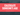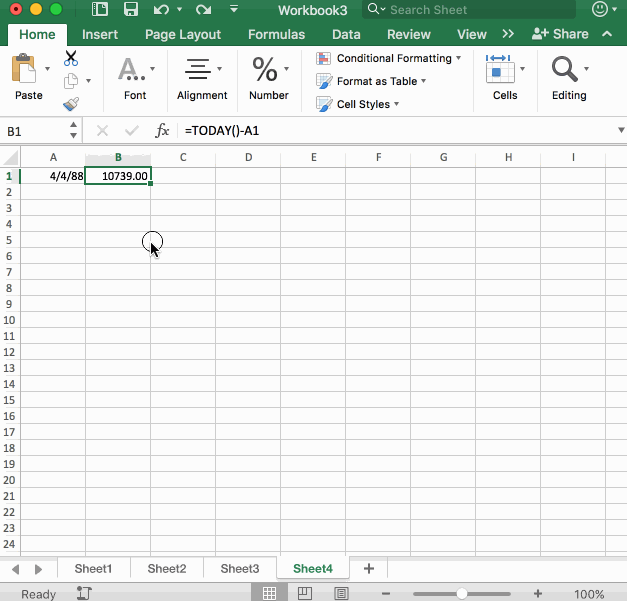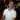# How to Calculate Someone's Age in Excel

August 08, 2017

Calculating someone’s age in Excel should be pretty straightforward. You would think there was an Age() function in Excel, but unfortunately, we’re on our own to figure this one out. No worries, though, it’s not as bad as you might think.

Let’s get started.

## The Problem

Say you have someone’s birthdate such as `4/4/1988`. How would you go about calculating their age?

You might say something like “easy, just take today’s date and subtract the birthdate.” Well, that’s a good starting point. Let’s work with that.

Assuming the birthdate is in `A1`, we’ll take today’s date (using the `TODAY()` function) and subtract the birthdate:

``````=TODAY()-\$A\$1
=5/5/29 (May 5th, 1929)``````

The result is May 5th, 1929? How did that happen?## How dates work in Excel

It’s important to take a second to explain how dates work in Excel. Put the number `1` in a cell and convert it to a date. It will display `1/1/1900`. This is because dates in Excel start from `1/1/1900`. If you changed the cell by putting a `2` in there, the date would become `1/2/1900`. What this means is that each number represents how many days it has been since `1/1/1900`.

Let’s look at the formula when we convert the dates into numbers.

``````=TODAY()-\$A\$1
=8/8/2017 - 4/4/1988
=42955 - 32237
=10718``````

If you put the number `10718` in a cell and format it as a date you’d get `5/5/29`.

But what does the number `10718` represent, anyway?

It actually represents the number of days between `4/4/1988` and `8/8/2017` (including leap days). So this person who has a birthdate of `4/4/1988` has lived for `10,718` days. We can use this to figure out how many years they are. We just need to divide by `365.25` (days in a year - including the fraction for leap years).

## Calculating Someone’s Age the Right Way

``````=(TODAY()-\$A\$1)/365.25
=29.3442847``````

This person is `29` years old. The fraction portion represents how much of a percentage of a new year they have lived. In our formula, this would be `~34%` of a new year, which translates to about `4` months. So `29` years and `4` months of age.

From here, we can either fix the number format to not include fractions or adjust the formula one last time:

``=ROUND((TODAY()-\$A\$1)/365.25,0)``

And that’s how you calculate someone’s age in Excel.

## But what if I want the EXACT Age?

What if you wanted to display someone’s age like the following:

``29 years 5 months and 2 days old``

How can we do this?

Let’s say in `A1` we have the following date:

``4/4/1988``

In cell `B1` we put the formula:

``=TODAY()-A1``

And then we convert the cell to a number format we get:

``10739``

What can we do with this?

Well, as an alternative to a special formula, we can use a Custom Number Format for the cell `B1`.

Right-Click the cell `B1` and select “Format Cell” then click on Custom and put in the following:

``y "years" m "months" d "days old"``In the custom number format, Excel understands when a number represents a date. We can use this to our advantage by utilizing the following custom number format info:

Text Meaning
y Year - Excel will take the number and get the amount of years that number would represent if that number were treated as a serial number.
m Month - Excel will figure out the amount of months (within a year) of the number if it were treated as a serial number.
d Day - Excel determines the amount of days (within a month) of the number if it were treated as a serial number.

To get a better understanding of how Excel Dates work, click here.

Wow, you read the whole article! You know, people who make it this far are true learners. And clearly, you value learning. Would you like to learn more about Excel? Please consider supporting me by buying me a coffee (it takes a lot of coffee to write these articles!).Written by Joseph who loves teaching about Excel.